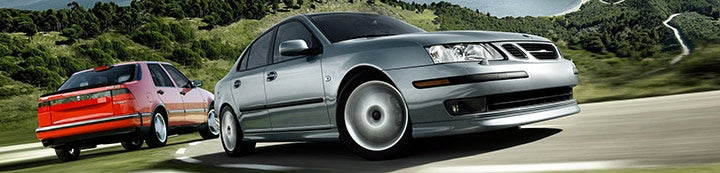1 - 3 of 3 Posts

#### California Saab Tuner

·
##### Registered
Joined
·
1,448 Posts
Discussion Starter · ·
I originally began doing some calculations a few hours ago to determine the tensile strength needed for a connecting rod in a Saab 2.3L.

I didn't have many exact numbers to start with but I estimated the piston + pin figure to be about a pound or so. Not including the connecting rod ...

First step:

I needed to create a formula to express the motion of the piston in the cyllinder. It's not a pure sin-like motion. Here's a picture showing the calculations:

The equation with the arrow pointed at it is the raw equation for expressing the motion of the piston in the cyllinder. "b" should actually be 1.77 however I also did an exagerrated calculation to show the effects of con rod ratio later and forgot to change the numbers back. If you use the equation to calculate the piston position/crank angle you'll need to use 1.77 as "b".

The equation makes a graph that looks vaguely like a cosine wave. However to show how it is slightly different I did an exagerrated version with a con rod ratio of .82 instead of 1.64. This smaller ratio shows how the wave is more narrow when the piston is closer to the top of the cyllinder. The solid line is the actual motion of the piston in a 2.3L Saab engine relative to crank angle:

The distance shown is in inches. It represents the total distance between the center of the crankshaft, and the center of the wrist pin.

The next image shows some of the calculations for finding the force on the con rod at certain points in the rotation. The differentiation for the motion equation, and speed equation, turned out to be so complex that the resulting equation took the calculator hours to solve. I decided just to pick a set of points and make the average accelleration from the average speed, and time difference:

The in cyllinder pressure is based off a non turbo 2.3L. I didn't have data for a turbo 2.3L and it would have depended on boost etc ... needless to say it will be higher during the combustion cycle in the turbo when under much boost.

Between the exhaust and intake stroke, when there is no combustion, there could be as much as about 1100 kg of force pulling up on the connecting rod!

Since the forces on the connecting rod vary more with RPM than pressure in the cyllinder, it would be wise to upgrade con rods when a higher rev-limit is going to be used.

Some examples of min cross section for various grades of metal assuming the afformentioned forces:

Titanium Ti-6Al-4V (Grade 5), STA Bar

Density: .16 lb/in
Tensile Strength, Yeild: 140,000 psi
Fatigue Strength: 102,000 psi
Min X section 6000+ infrequent: .021 square inches
Min X section 6000+ frequent: .029 square inches

VascoMax? C-350 Specialty Steel, Heat Treatment: 927?C (1700?F) + Age

Density: 0.292 lb/in
Tensile Strength, Yeild: 325,000 psi
Fatigue Strength: not available
Min X section 6000+ infrequent: .0092 square inches
Min X section 6000+ frequent: .0092 square inches

Aluminum 7178-T76; 7178-T7651

Density: 0.102 lb/in
Tensile Strength, Yeild: 73,200 psi
Fatigue Strength: 23,200 psi
Min X section 6000+ infrequent: .041 square inches
Min X section 6000+ frequent: .129 square inches

Of course all of those cross sectional areas are much less than you'd actually use as you expect vibration and sudden impact to greatly affect strength. They should however give a good idea what sort of strengths are required.

All of the above metals are also WELL outside the grades usually used in cars. They are aerospace quality metals. In some cases they are easily twice the strength of the same metal used in automotive applications. Here is where I got the information from.

Hope this post has been usefull as it was a bit long.
Anyone who read to the end deserves some kind of prize I think.

Cheers,
Dubbya

#### sj

·
##### Registered
Joined
·
836 Posts
I got there and I claim my prize, please
What is it by the way?

It would be nice to know the specification of the steel (or whatever it is) which is actually used by Saab, wouldn't it?

#### California Saab Tuner

·
##### Registered
Joined
·
1,448 Posts
Discussion Starter · ·
Most forged steel alloys are around the 450 MPa range for tensile strength. Saab probably uses something about there. The steel I listed is an aerospace maraging alloy! It's the highest tensile strength they had. It had a tensile strength of about 2100 MPa ... or about 4.7 times stronger than typical forged steel!

Dubbya

btw the prize is ummm ... ok so I lied about the prize. But no one will know that until they read it all. *insert evil laugh here*

1 - 3 of 3 Posts